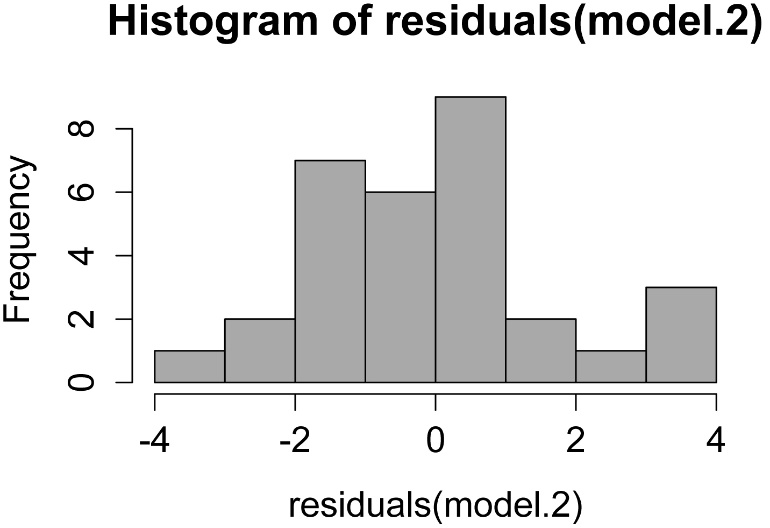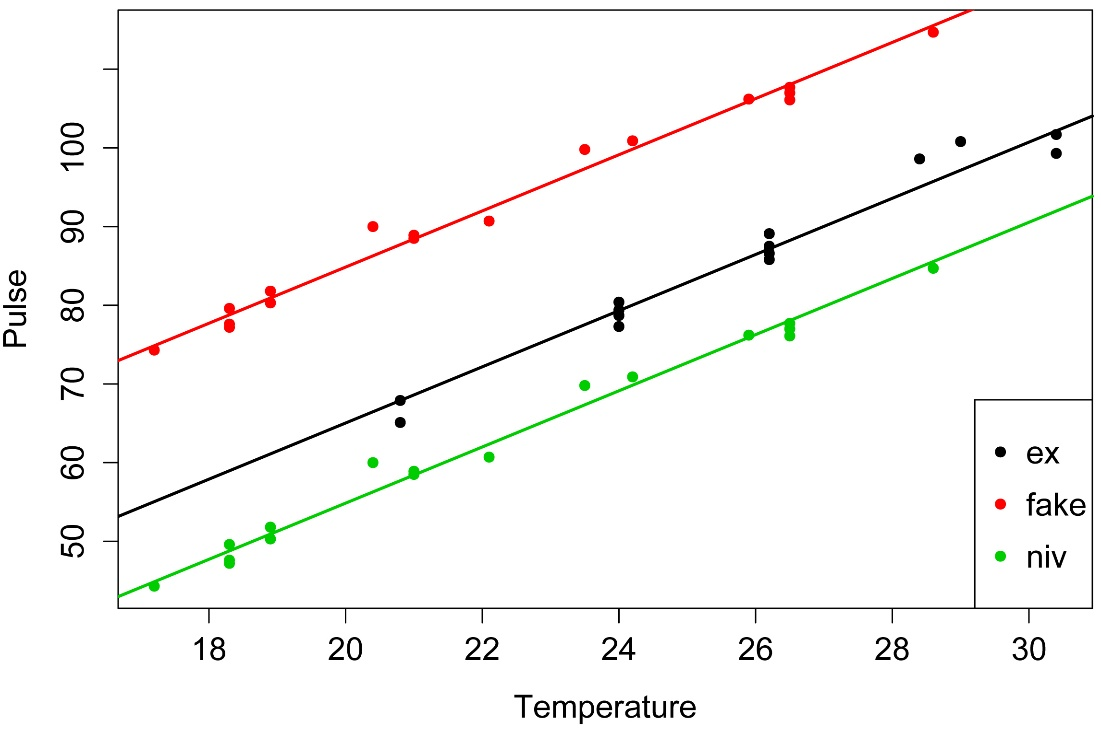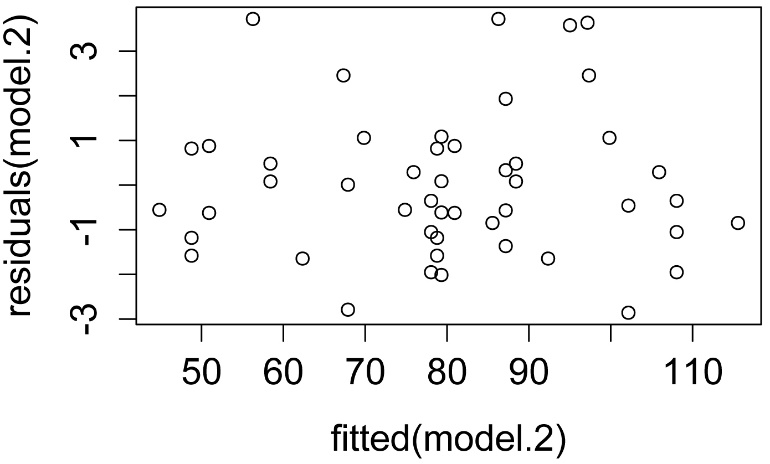## An R Companion for the Handbook of Biological Statistics

Salvatore S. Mangiafico

# Analysis of Covariance

When to use it

The cricket example is shown in the “How to do the test” section.

Null hypotheses

Assumptions

How the test works

Examples

Graphing the results

Similar tests

See the Handbook for information on these topics.

### How to do the test

#### Analysis of covariance example with two categories and type II sum of squares

This example uses type II sum of squares, but otherwise follows the example in the Handbook.  The parameter estimates are calculated differently in R, so the calculation of the intercepts of the lines is slightly different.

### --------------------------------------------------------------
### Analysis of covariance, cricket example
### pp. 228–229
### --------------------------------------------------------------

Input = ("
Species   Temp   Pulse
ex       20.8   67.9
ex       20.8   65.1
ex       24     77.3
ex       24     78.7
ex       24     79.4
ex       24     80.4
ex       26.2   85.8
ex       26.2   86.6
ex       26.2   87.5
ex       26.2   89.1
ex       28.4   98.6
ex       29    100.8
ex       30.4   99.3
ex       30.4  101.7
niv      17.2   44.3
niv      18.3   47.2
niv      18.3   47.6
niv      18.3   49.6
niv      18.9   50.3
niv      18.9   51.8
niv      20.4   60
niv      21     58.5
niv      21     58.9
niv      22.1   60.7
niv      23.5   69.8
niv      24.2   70.9
niv      25.9   76.2
niv      26.5   76.1
niv      26.5   77
niv      26.5   77.7
niv      28.6   84.7
")

##### Simple plot

plot(x   = Data\$Temp,
y   = Data\$Pulse,
col = Data\$Species,
pch = 16,
xlab = "Temperature",
ylab = "Pulse")

legend('bottomright',
legend = levels(Data\$Species),
col = 1:2,
cex = 1,
pch = 16)

##### Analysis of covariance

options(contrasts = c("contr.treatment", "contr.poly"))

### These are the default contrasts in R

model.1 = lm (Pulse ~ Temp + Species + Temp:Species,
data = Data)

library(car)

Anova(model.1, type="II")

Anova Table (Type II tests)

Sum Sq Df  F value    Pr(>F)

Temp         4376.1  1 1388.839 < 2.2e-16 ***

Species       598.0  1  189.789 9.907e-14 ***

Temp:Species    4.3  1    1.357    0.2542

### Interaction is not significant, so the slope across groups

### is not different.

model.2 = lm (Pulse ~ Temp + Species,
data = Data)

library(car)

Anova(model.2, type="II")

Anova Table (Type II tests)

Sum Sq Df F value    Pr(>F)

Temp      4376.1  1  1371.4 < 2.2e-16 ***

Species    598.0  1   187.4 6.272e-14 ***

### The category variable (Species) is significant,

### so the intercepts among groups are different

summary(model.2)

Coefficients:

Estimate Std. Error t value Pr(>|t|)

(Intercept)  -7.21091    2.55094  -2.827  0.00858 **

Temp          3.60275    0.09729  37.032  < 2e-16 ***

Speciesniv  -10.06529    0.73526 -13.689 6.27e-14 ***

### Note that these estimates are different than in the Handbook,

###   but the calculated results will be identical.

### The slope estimate is the same.

### The intercept for species 1 (ex) is (intercept).

### The intercept for species 2 (niv) is (intercept) + Speciesniv.

### This is determined from the contrast coding of the Species

### variable shown below, and the fact that Speciesniv is shown in

### coefficient table above.

contrasts(Data\$Species)

niv

ex    0

niv   1

##### Simple plot with fitted lines

I.nought = -7.21091
I1 = I.nought + 0
I2 = I.nought + -10.06529
B  = 3.60275

plot(x   = Data\$Temp,
y   = Data\$Pulse,
col = Data\$Species,
pch = 16,
xlab = "Temperature",
ylab = "Pulse")

legend('bottomright',
legend = levels(Data\$Species),
col = 1:2,
cex = 1,
pch = 16)

abline(I1, B,
lty=1, lwd=2, col = 1)

abline(I2, B,
lty=1, lwd=2, col = 2)##### p-value and R-squared of combined model

summary(model.2)

Multiple R-squared:  0.9896,  Adjusted R-squared:  0.9888

F-statistic:  1331 on 2 and 28 DF,  p-value: < 2.2e-16

##### Checking assumptions of the model

hist(residuals(model.2),
col="darkgray")A histogram of residuals from a linear model.  The distribution of these residuals should be approximately normal.

plot(fitted(model.2),
residuals(model.2))A plot of residuals vs. predicted values.  The residuals should be unbiased and homoscedastic.  For an illustration of these properties, see this diagram by Steve Jost at DePaul University: condor.depaul.edu/sjost/it223/documents/resid-plots.gif.

### additional model checking plots with: plot(model.2)
### alternative: library(FSA); residPlot(model.2)

#     #     #

#### Analysis of covariance example with three categories and type II sum of squares

This example uses type II sum of squares, and considers a case with three groups.

### --------------------------------------------------------------
### Analysis of covariance, hypothetical data
### --------------------------------------------------------------

Input = ("
Species   Temp   Pulse
ex       20.8   67.9
ex       20.8   65.1
ex       24     77.3
ex       24     78.7
ex       24     79.4
ex       24     80.4
ex       26.2   85.8
ex       26.2   86.6
ex       26.2   87.5
ex       26.2   89.1
ex       28.4   98.6
ex       29    100.8
ex       30.4   99.3
ex       30.4  101.7
niv      17.2   44.3
niv      18.3   47.2
niv      18.3   47.6
niv      18.3   49.6
niv      18.9   50.3
niv      18.9   51.8
niv      20.4   60
niv      21     58.5
niv      21     58.9
niv      22.1   60.7
niv      23.5   69.8
niv      24.2   70.9
niv      25.9   76.2
niv      26.5   76.1
niv      26.5   77
niv      26.5   77.7
niv      28.6   84.7
fake     17.2   74.3
fake     18.3   77.2
fake     18.3   77.6
fake     18.3   79.6
fake     18.9   80.3
fake     18.9   81.8
fake     20.4   90
fake     21     88.5
fake     21     88.9
fake     22.1   90.7
fake     23.5   99.8
fake     24.2   100.9
fake     25.9   106.2
fake     26.5   106.1
fake     26.5   107
fake     26.5   107.7
fake     28.6   114.7
")

##### Simple plot

plot(x   = Data\$Temp,
y   = Data\$Pulse,
col = Data\$Species,
pch = 16,
xlab = "Temperature",
ylab = "Pulse")

legend('bottomright',
legend = levels(Data\$Species),
col = 1:3,
cex = 1,
pch = 16)

##### Analysis of covariance

options(contrasts = c("contr.treatment", "contr.poly"))

### These are the default contrasts in R

model.1 = lm (Pulse ~ Temp + Species + Temp:Species,
data = Data)

library(car)

Anova(model.1, type="II")

Sum Sq Df   F value Pr(>F)

Temp         7026.0  1 2452.4187 <2e-16 ***

Species      7835.7  2 1367.5377 <2e-16 ***

Temp:Species    5.2  2    0.9126 0.4093

### Interaction is not significant, so the slope among groups

### is not different.

model.2 = lm (Pulse ~ Temp + Species,
data = Data)

library(car)

Anova(model.2, type="II")

Sum Sq Df F value    Pr(>F)

Temp      7026.0  1  2462.2 < 2.2e-16 ***

Species   7835.7  2  1373.0 < 2.2e-16 ***

Residuals  125.6 44

### The category variable (Species) is significant,

### so the intercepts among groups are different

summary(model.2)

Coefficients:

Estimate Std. Error t value Pr(>|t|)

(Intercept)  -6.35729    1.90713  -3.333  0.00175 **

Temp          3.56961    0.07194  49.621  < 2e-16 ***

Speciesfake  19.81429    0.66333  29.871  < 2e-16 ***

Speciesniv  -10.18571    0.66333 -15.355  < 2e-16 ***

### The slope estimate is the Temp coefficient.

### The intercept for species 1 (ex) is (intercept).

### The intercept for species 2 (fake) is (intercept) + Speciesfake.

### The intercept for species 3 (niv) is (intercept) + Speciesniv.

### This is determined from the contrast coding of the Species

### variable shown below.

contrasts(Data\$Species)

fake niv

ex      0   0

fake    1   0

niv     0   1

##### Simple plot with fitted lines

I.nought = -6.35729
I1 = I.nought + 0
I2 = I.nought + 19.81429
I3 = I.nought + -10.18571
B  = 3.56961

plot(x   = Data\$Temp,
y   = Data\$Pulse,
col = Data\$Species,
pch = 16,
xlab = "Temperature",
ylab = "Pulse")

legend('bottomright',
legend = levels(Data\$Species),
col = 1:3,
cex = 1,
pch = 16)

abline(I1, B,
lty=1, lwd=2, col = 1)

abline(I2, B,
lty=1, lwd=2, col = 2)

abline(I3, B,
lty=1, lwd=2, col = 3)##### p-value and R-squared of combined model

summary(model.2)

Multiple R-squared:  0.9919,  Adjusted R-squared:  0.9913

F-statistic:  1791 on 3 and 44 DF,  p-value: < 2.2e-16

##### Checking assumptions of the model

hist(residuals(model.2),
col="darkgray")A histogram of residuals from a linear model.  The distribution of these residuals should be approximately normal.

plot(fitted(model.2),
residuals(model.2))A plot of residuals vs. predicted values.  The residuals should be unbiased and homoscedastic.  For an illustration of these properties, see this diagram by Steve Jost at DePaul University: condor.depaul.edu/sjost/it223/documents/resid-plots.gif.

### additional model checking plots with: plot(model.2)
### alternative: library(FSA); residPlot(model.2)

#     #     #

Power analysis

See the Handbook for information on this topic.